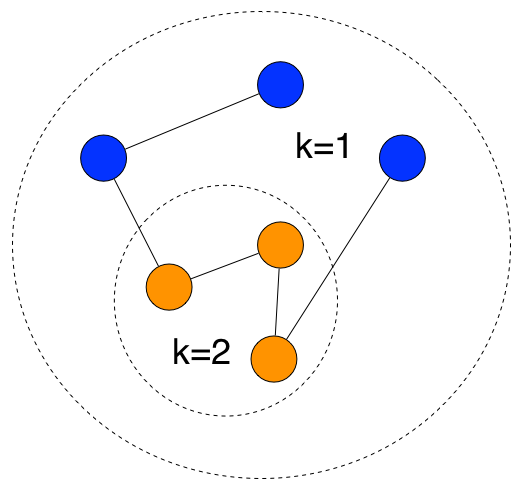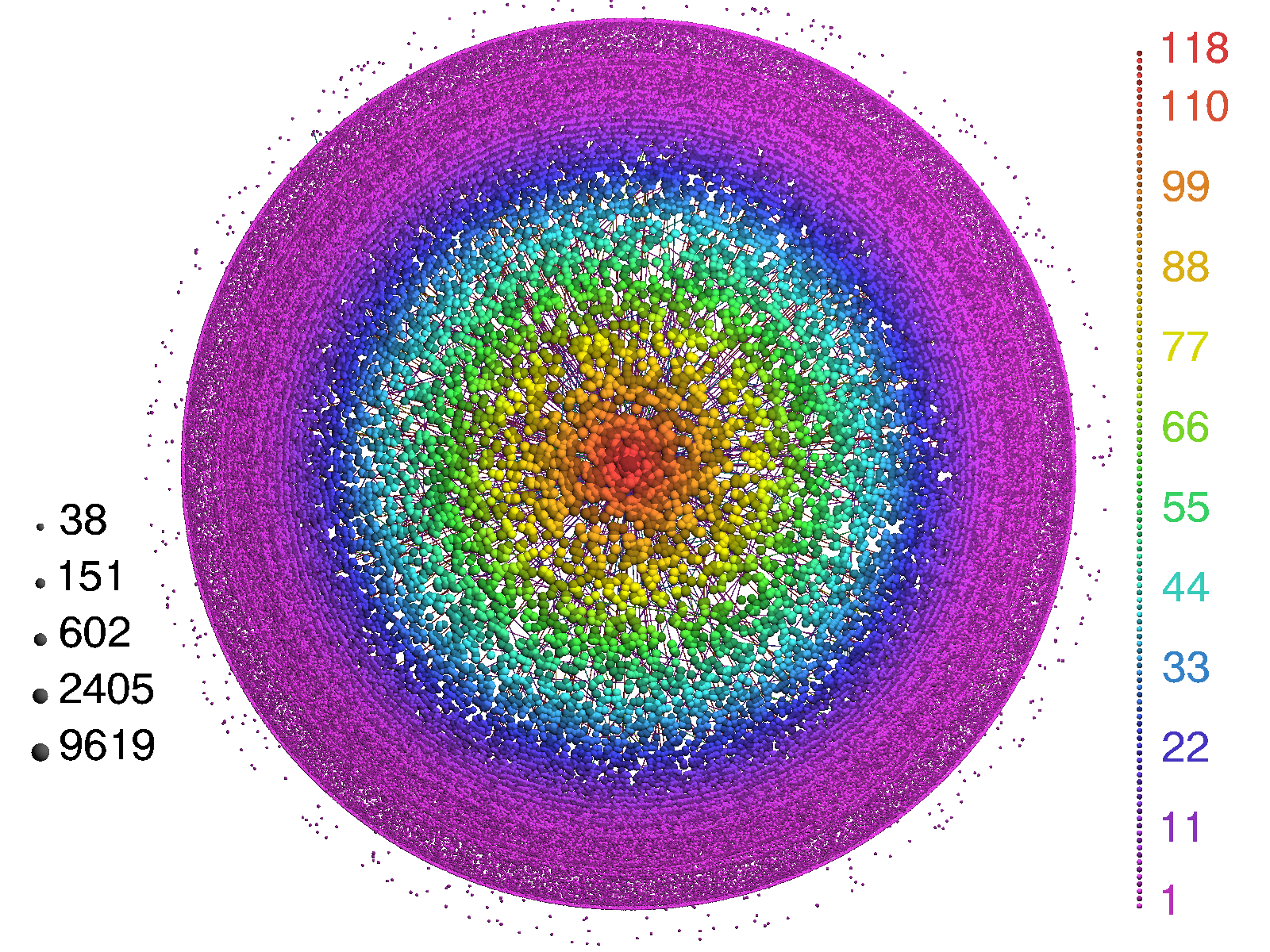# Visualizing The Structure Of An Occupation Network

In a previous post, I presented an analysis of an occupation network and how one might use a particular measure of network structure, eigenvector centrality, to identify important occupation titles. Here, I will present a visual method for performing the same task.

## What is an occupation network?

An occupation network is comprised of a set of occupation titles that are connected to one another. Titles are connected when a person transitions between them, for example through a career change or job promotion. Using this definition of connectedness and data from nearly 1,000,000 publicly available resumes, I built the network by extracting and interconnecting occupation titles according the job transitions recorded in the resumes. The network consists of roughly 300,000 vertices and 1,500,000 edges.

## K-core decomposition

K-core decomposition is a method for breaking a network up into sets, or shells, according to degree. If we define a network as $G = (V,E)$, where $V$ is the set of vertices and $E$ is the set of edges inter-connecting them, then a k-core is the subset of vertices in $V$ with degree (total number of edges) $\ge k$. The decomposition works by recursively removing vertices from the network that have a degree less than $k$. The remaining vertices are placed in a set and the process is repeated until $k=1$. The figure below shows a simple example.Each set of vertices are then plotted in a circular fashion, according to geometric layout algorithm. Vertices with largest $k$ are placed in the middle of the figure and vertices with decreasing $k$ are placed further away from the middle, using a logarithmic scaling factor. The resulting plot allows for the identification of hierarchical structure and important vertices, according to their degree centrality. A detailed explanation of the algorithm can be found here. The software, Lanet, used to generate the visualization contained in this post can be found here.

#### Visualizing the network

The figure below presents a visualization (showing 1% of vertices) of the k-core decomposition of the occupation network. According to right-hand legend, the colors correspond to the size of k. Purple maps to $k = 1$ and red maps to $k \ge 118$.What can we learn about the network from the visualization? The most prominent feature is the hierarchical arrangement of distinct sets of colored vertices. Most occupation titles in the network have only a single edge, while a select few have 118 or more.

From a career path perspective, red colored occupation titles offer the most diverse set of next moves and also provide a central position within the network. Such a position offers relatively short paths to any other occupation title, when compared to vertices with lower degrees. For workers unsure of where to go next in their careers, targeting these central vertices as next positions provides the largest number of immediate future opportunities than others.

Which occupation titles are most central? For a list of the top 10, see this post.

complex networks

Archives